#### 期刊菜单

Lump Solutions and Mixed Lump-Stripe Solutions of a (2 + 1)-Dimensional KdV Equation
DOI: 10.12677/MP.2021.116017, PDF, HTML, XML, 下载: 130  浏览: 254  科研立项经费支持

Abstract: In this paper, by using Hirota bilinear form, the lumped solution and lump strip mixed solution of (2 + 1)-dimensional KdV equation are obtained with the help of Maple symbolic calculation in this paper. By choosing different parameters, the dynamic characteristics of these solutions are studied combined with images, especially the interaction between lumps and stripe solutions. These solutions and their related properties will help to study the dynamic characteristics described by physical phenomena of the (2 + 1)-dimensional KdV equation.

1. 引言

${D}_{t}^{m}{D}_{x}^{n}\left(f\cdot f\right)={D}_{t}^{m}{D}_{x}^{n}\left(f\left(t,x,y\right)f\left(t,x,y\right)\right)=\frac{{\partial }^{m}}{\partial {T}^{n}}\frac{{\partial }^{n}}{\partial {T}^{n}}×{\left[f\left(t+T,x+X,y\right)f\left(t-T,x-X,y\right)\right]|}_{T=0,X=0}.$

${u}_{t}+3u{u}_{y}+{u}_{xxy}+3{u}_{x}{\int }_{-\infty }^{x}{u}_{y}\left(t,x,y\right)\text{d}x=0$ (1)

2. (2 + 1)-维KdV方程的lump解与lump-stripe解

$2{\left(\mathrm{ln}f\right)}_{xxt}+6\left[{u}_{0}+2{\left(\mathrm{ln}f\right)}_{xx}\right]{\left(\mathrm{ln}f\right)}_{xxy}+2{\left(\mathrm{ln}f\right)}_{xxxxy}+12{\left(\mathrm{ln}f\right)}_{xxx}{\left(\mathrm{ln}f\right)}_{xy}=0.$ (2)

${D}_{x}\left({D}_{t}+3{u}_{0}{D}_{y}+{D}_{x}^{2}{D}_{y}\right)\left(f\cdot f\right)=0.$ (3)

$f={g}^{2}+{h}^{2}+{k}_{1}{\text{e}}^{\left({k}_{2}x+{k}_{3}y+{k}_{4}t\right)}+{a}_{9}$ (4)

(1) 当 ${k}_{1}=0$ 时，可以得到方程(1)的若干种不同形式的lump解。在此，只讨论其中的一种：

${a}_{1}={a}_{1},{a}_{2}=0,{a}_{3}=0,{a}_{4}={a}_{4},{a}_{5}=0,{a}_{6}={a}_{6},{a}_{7}={a}_{7},{a}_{8}={a}_{8},{a}_{9}={a}_{9},{u}_{0}=-\frac{{a}_{7}}{3{a}_{6}}.$ (5)

$f={\left({a}_{1}x+{a}_{4}\right)}^{2}+{\left({a}_{6}y+{a}_{7}t+{a}_{8}\right)}^{2}+{a}_{9}.$ (6)

$u={u}_{0}+\frac{4{a}_{1}^{2}f-8{a}_{1}^{2}{g}^{2}}{{f}^{2}}.$ (7)

$\left({x}_{1},{y}_{1}\right)=\left(-\frac{{a}_{4}}{{a}_{1}},-\frac{{a}_{7}t+{a}_{8}}{{a}_{6}}\right)$

$\Delta ={|\begin{array}{cc}\frac{{\partial }^{2}u}{\partial {x}^{2}}& \frac{{\partial }^{2}u}{\partial x\partial y}\\ \frac{{\partial }^{2}u}{\partial x\partial y}& \frac{{\partial }^{2}u}{\partial {y}^{2}}\end{array}|}_{\left(-\frac{{a}_{4}}{{a}_{1}},-\frac{{a}_{7}t+{a}_{8}}{{a}_{6}}\right)}=\frac{192{a}_{1}^{6}{a}_{6}^{2}}{{a}_{9}^{4}}>0.$ (8)

${u}_{xx}\left({x}_{1},{y}_{1}\right)=-\frac{24{a}_{1}^{4}}{{a}_{9}^{2}}<0$，因此式(7)在 $\left({x}_{1},{y}_{1}\right)$ 处得到最大值，且最大值为 $-\frac{{a}_{7}}{3{a}_{6}}+\frac{4{a}_{1}^{2}}{{a}_{9}}$。当参数

${a}_{1}=1,{a}_{4}=1,{a}_{6}=-1,{a}_{7}=2,{a}_{8}=1,{a}_{9}=2$ 时，式(7)的lump解的在 $t=-3,t=0,t=3$ 三个不同时刻的三维图和密度图如图所示：

(2) 当 ${k}_{1}\ne 0$ 时，下面讨论双线性方程(3)的四种lump-stripe解。

$\begin{array}{l}{k}_{1}={k}_{1},{k}_{2}=0,{k}_{3}={k}_{3},{k}_{4}=0,{a}_{1}=0,{a}_{2}={a}_{2},{a}_{3}=0,\\ {a}_{4}={a}_{4},{a}_{5}={a}_{5},{a}_{6}=0,{a}_{7}=0,{a}_{8}={a}_{8},{a}_{9}={a}_{9},{u}_{0}=0.\end{array}$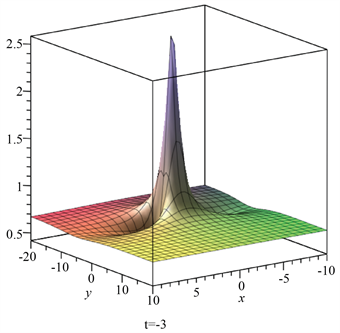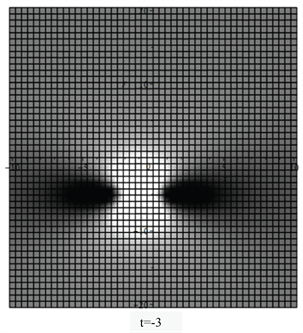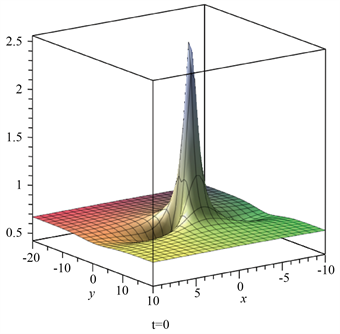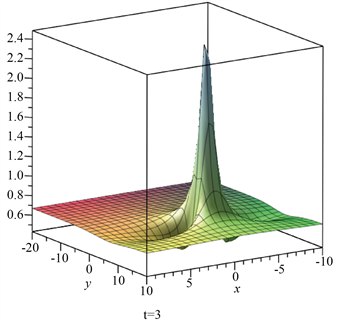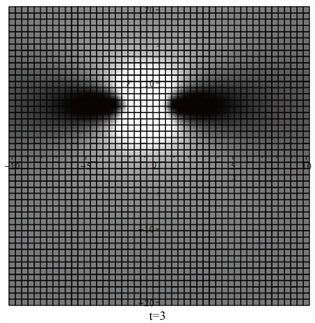Figure 1. Three dimensional diagrams and density diagrams of equation (7) at different times

$f={\left({a}_{2}y+{a}_{4}\right)}^{2}+{\left({a}_{5}x+{a}_{8}\right)}^{2}+{k}_{1}{\text{e}}^{{k}_{3}y}+{a}_{9}.$ (9)

${k}_{1}=1,{k}_{3}=1,{a}_{2}=1,{a}_{4}=2,{a}_{5}=1,{a}_{8}=1,{a}_{9}=2,{u}_{0}=0$

$u=\frac{4}{{\left(y+2\right)}^{2}+{\left(x+1\right)}^{2}+{\text{e}}^{y}+2}-\frac{8{\left(x+1\right)}^{2}}{{\left({\left(y+2\right)}^{2}+{\left(x+1\right)}^{2}+{\text{e}}^{y}+2\right)}^{2}}.$ (10)Figure 2. Three dimensional diagrams and density diagrams of equation (10)

$\begin{array}{l}{k}_{1}={k}_{1},{k}_{2}=0,{k}_{3}={k}_{3},{k}_{4}={k}_{4},{a}_{1}=0,{a}_{2}=\frac{{k}_{3}{a}_{3}}{{k}_{4}},{a}_{3}={a}_{3},\\ {a}_{4}={a}_{4},{a}_{5}={a}_{5},{a}_{6}=0,{a}_{7}=0,{a}_{8}={a}_{8},{a}_{9}={a}_{9},{u}_{0}=-\frac{{k}_{4}}{3{k}_{3}}.\end{array}$

$f={\left(\frac{{k}_{3}{a}_{3}}{{k}_{4}}y+{a}_{3}t+{a}_{4}\right)}^{2}+{\left({a}_{5}x+{a}_{8}\right)}^{2}+{k}_{1}{\text{e}}^{{k}_{3}y+{k}_{4}t}+{a}_{9}.$ (11)

${k}_{1}=1,{k}_{3}=1,{k}_{4}=2,{a}_{3}=1,{a}_{4}=2,{a}_{5}=1,{a}_{8}=1,{a}_{9}=2$

$u=-\frac{2}{3}+\frac{4}{{\left(0.5y+t+2\right)}^{2}+{\left(x+1\right)}^{2}+{\text{e}}^{y+2t}+2}-\frac{8{\left(x+1\right)}^{2}}{{\left({\left(0.5y+t+2\right)}^{2}+{\left(x+1\right)}^{2}+{\text{e}}^{y+2t}+2\right)}^{2}}.$ (12)

$\begin{array}{l}{k}_{1}={k}_{1},{k}_{2}=0,{k}_{3}={k}_{3},{k}_{4}={k}_{4},{a}_{1}={a}_{1},{a}_{2}=-\frac{{a}_{5}{a}_{6}}{{a}_{1}},{a}_{3}=-\frac{{k}_{4}{a}_{5}{a}_{6}}{{k}_{3}{a}_{1}},\\ {a}_{4}={a}_{4},{a}_{5}={a}_{5},{a}_{6}={a}_{6},{a}_{7}=\frac{{k}_{4}{a}_{6}}{{k}_{3}},{a}_{8}={a}_{8},{a}_{9}={a}_{9},{u}_{0}=-\frac{{k}_{4}}{3{k}_{3}}.\end{array}$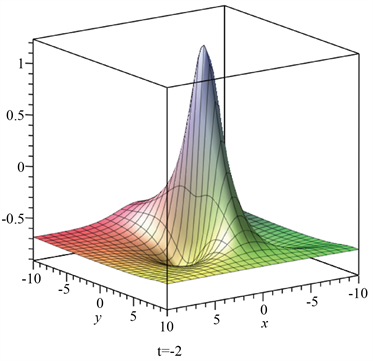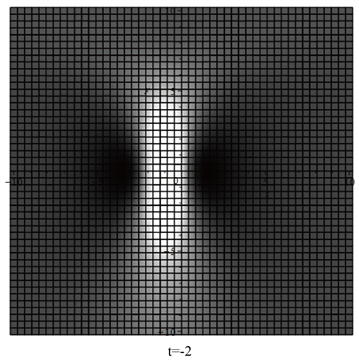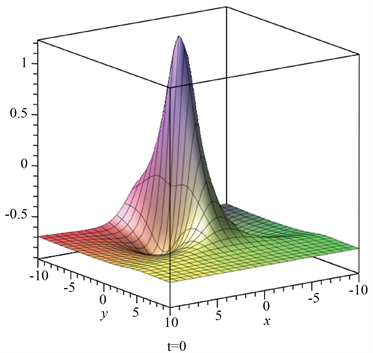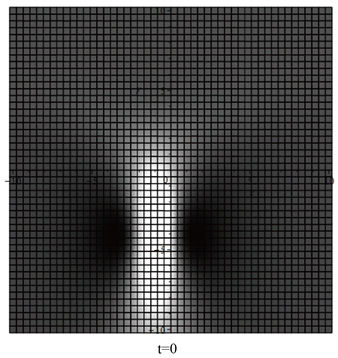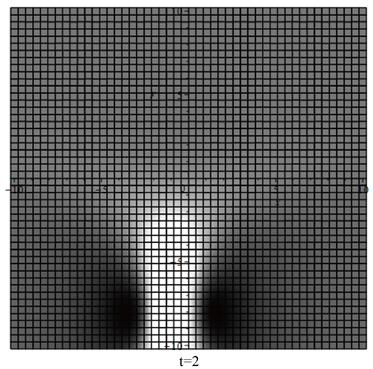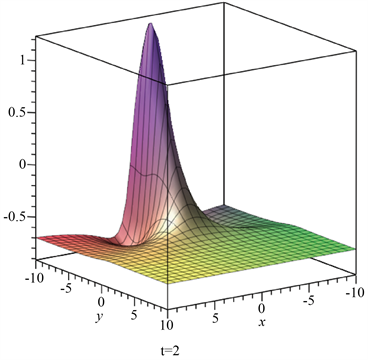Figure 3. Three dimensional diagrams and density diagrams of equation (12) at different times

$f={\left({a}_{1}x--\frac{{a}_{5}{a}_{6}}{{a}_{1}}y-\frac{{k}_{4}{a}_{5}{a}_{6}}{{k}_{3}{a}_{1}}t+{a}_{4}\right)}^{2}+{\left({a}_{5}x+{a}_{6}y+\frac{{k}_{4}{a}_{6}}{{k}_{3}}t+{a}_{8}\right)}^{2}+{k}_{1}{\text{e}}^{{k}_{3}y+{k}_{4}t}+{a}_{9}.$ (13)

${k}_{1}=1,{k}_{3}=2,{k}_{4}=-1,{a}_{1}=2,{a}_{4}=1,{a}_{5}=1,{a}_{6}=2,{a}_{8}=1,{a}_{9}=1$

$\begin{array}{c}u=\frac{1}{6}+\frac{20}{{\left(2x-y+0.5t+1\right)}^{2}+{\left(x+2y-t+1\right)}^{2}+{\text{e}}^{2y-t}+1}\\ -\frac{8{\left(5x+3\right)}^{2}}{{\left({\left(2x-y+0.5t+1\right)}^{2}+{\left(x+2y-t+1\right)}^{2}+{\text{e}}^{2y-t}+1\right)}^{2}}.\end{array}$ (14)

$\begin{array}{l}{a}_{1}=0,{a}_{2}=0,{a}_{3}=0,{a}_{4}={a}_{4},{a}_{5}=0,{a}_{6}={a}_{6},{a}_{7}={a}_{7},{a}_{8}={a}_{8},\\ {a}_{9}={a}_{9},{k}_{1}={k}_{1},{k}_{2}={k}_{2},{k}_{3}={k}_{3},{k}_{4}=\frac{{a}_{7}{k}_{3}}{{a}_{6}},{u}_{0}=-\frac{{a}_{6}{k}_{2}^{2}+{a}_{7}}{3{a}_{6}}.\end{array}$

$f={a}_{4}^{2}+{\left({a}_{6}y+{a}_{7}t+{a}_{8}\right)}^{2}+{k}_{1}{\text{e}}^{{k}_{2}x+{k}_{3}y+\frac{{a}_{7}{k}_{3}}{{a}_{6}}t}+{a}_{9}.$ (15)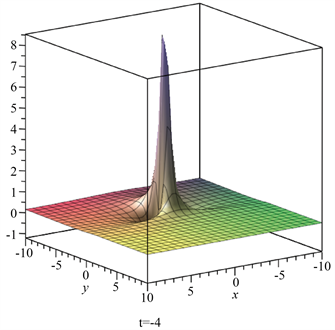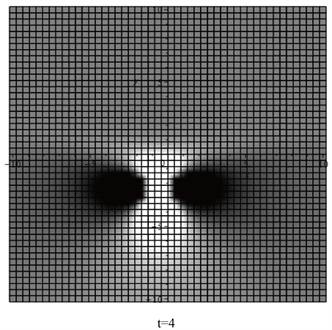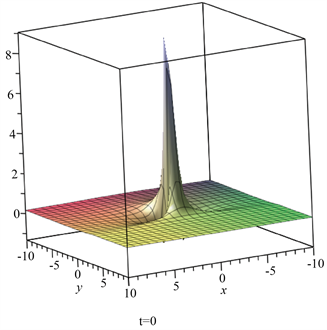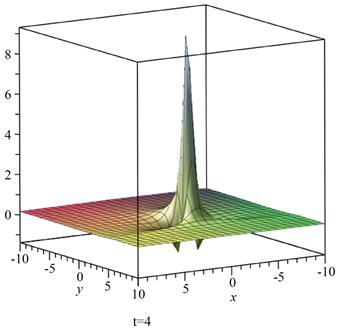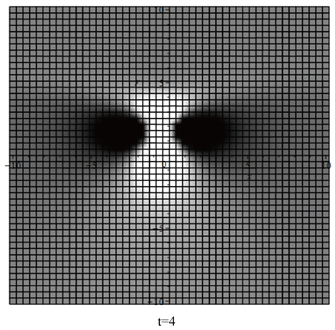Figure 4. Three dimensional diagrams and density diagrams of equation (14) at different times

${a}_{4}=1,{a}_{6}=1,{a}_{7}=2,{a}_{8}=2,{a}_{9}=1,{k}_{1}=1,{k}_{2}=2,{k}_{3}=-1$

$u=-1+\frac{8{\text{e}}^{2x-y-2t}}{{\left(y+2t+2\right)}^{2}+{\text{e}}^{2x-y-2t}+2}-\frac{8{\left({\text{e}}^{2x-y-2t}\right)}^{2}}{{\left({\left(y+2t+2\right)}^{2}+{\text{e}}^{2x-y-2t}+2\right)}^{2}}.$ (16)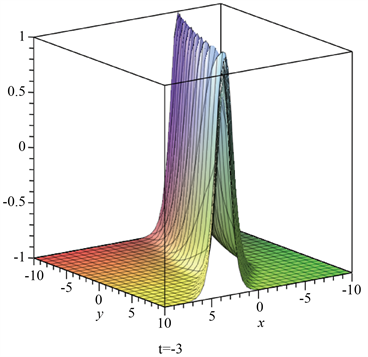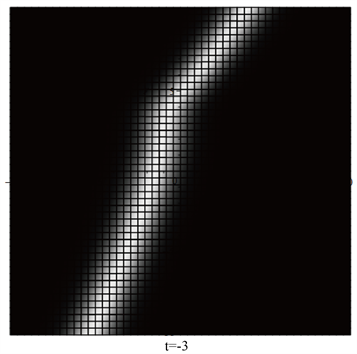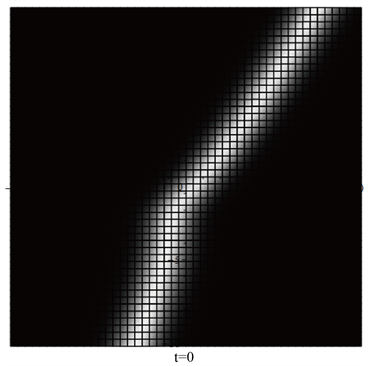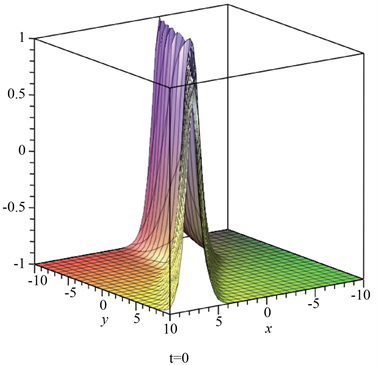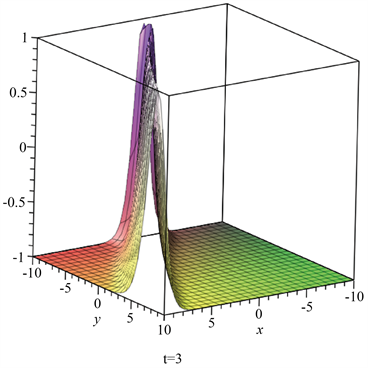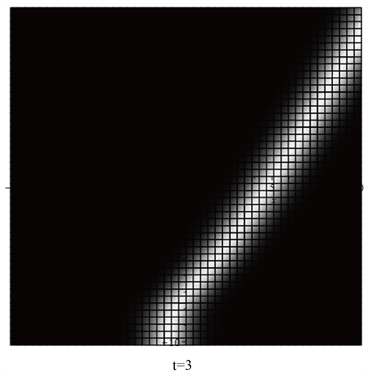Figure 5. Three dimensional diagrams and density diagrams of equation (16) at different times

3. 结束语

Hirota双线性方法是求可积方程孤子解的一种非常有效的方法。本文直接利用双线性方法，借助Maple符号计算软件，得到了(2 + 1)维KdV方程的lump解及lump-stripe混合解。并且借助符号计算软件Maple将本文中获得的所有解都代入方程(1)中进行了检验。本文所得到的四种lump-stripe混合解各有特点：第一种混合解不显含时间t；第二种与第三种混合解中lump孤子与stripe孤子发生的是弹性碰撞，并且lump孤子吞噬了stripe孤子；第四种混合解中lump孤子与stripe孤子发生的是非弹性碰撞，并且lump孤子与stripe孤子一直结合在一块，形状不随时间发生改变。这些结果对于理解(2 + 1)维KdV方程所描述的物理现象具有一定的理论参考价值。另外，本文求非线性演化方程lump解及lump-stripe混合解的方法简单有效，可以推广到其它一系列的非线性偏微分方程的求解。

  Hirota, R. (2004) The Direct Method in Soliton Theory. Cambridge University Press, Cambridge, England‎. https://doi.org/10.1017/CBO9780511543043  Zhang, Y.-F., Tam, H. and Zhao, J. (2006) Higher-Dimensional KdV Equations and Their Soliton Solutions. Communications in Theoretical Physics, 45, 411-413. https://doi.org/10.1088/0253-6102/45/3/007  冯滨鲁, 郭婷婷. (2 + 1)维KdV方程的Gramm解及其pfaffian化[J]. 潍坊学院学报, 2008, 8(6): 75-78.  Ma, W.X. (2015) Lump Solutions to the Kadomtsev-Petviashvili Equa-tion. Physics Letters A, 379, 1975-1978. https://doi.org/10.1016/j.physleta.2015.06.061  Zhao, Z.L., Chen, Y. and Han, B. (2017) Lump Soliton, Mixed Lump Tripe and Periodic Lump Solutions of a (2 + 1)-Dimensional Asymmetrical Nizhnik-Novikov-Veselov Equation. Modern Physics Letters B, 31, 1750157. https://doi.org/10.1142/S0217984917501573  Chen, S.T. and Ma, W.X. (2018) Lump Solutions to a Genera-lized Bogoyavlensky-Konopelchenko Equation. Frontiers of Mathematics in China, 13, 525-534. https://doi.org/10.1007/s11464-018-0694-z  Chen, S.T. and Ma, W.X. (2018) Lump Solutions of a Generalized Calogero-Bogoyavlenskii-Schiff Equation. Computers & Mathematics with Applications, 76, 1680-1685. https://doi.org/10.1016/j.camwa.2018.07.019  Zhang, Y.F., Ma, W.X. and Yang, J.Y. (2020) A Study on Lump Solutions to a (2 + 1)-Dimensional Completely Generalized Hirota-Satsuma-Ito Equation. Discrete and Conti-nuous Dynamical Systems, Series S, 13, 2941-2948. https://doi.org/10.3934/dcdss.2020167